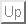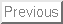Math 701:
Calculus of Variations and Tensor Calculus

PART I: CALCULUS OF VARIATIONS

Lecture 1

Chapter I: Fundamental Ideas

1. Multivariable Calculus as a Prelude to Calculus of Variations
2. Some Simple Problems in Calculus of Variations
3. Methods for Solving Problems in Calculus of Variations
1. Method of Finite Differences

Lecture 2
2. Method of Variations
1. Variants and Variations
2. Variational Derivative
3. Euler's Differential Equation
3. Solved Example

Lecture 3
4. Integration of Euler's Differential Equation

Chapter II: Generalizations

1. Functional with Several Unknown Functions

Lecture 4
2. Extremum Problems with Side Conditions
1. Isoperimetric Problems
1. Heuristic Solution
2. Solution via Constraint Manifold

Lecture 5
2. Variational Problems with Finite Constraints
3. Variable End Point Problem
4. Extremum Problem at a Moment of Time Symmetry

Lecture 6

Generic Variable Endpoint Problem

1. General Variations in the Functional
2. Transversality Conditions
3. Junction Conditions

Lecture 7
Parametrization Invariant Problem
1. Variational Principle for a Geodesic

Lecture 8
2. Equation of Geodesic Motion
1. Parametric Invariance
2. Parametrization in Terms of Curve Length

Lecture 9
3. Physical Significance of the Equation for a Geodesic
4. Equivalence Principle and ``Gravitation''=``Geometry''

Lecture 10

Chapter III: Variational Formulation of Mechanics

1. Hamilton's Principle

Lecture 11
2. Hamilton-Jacobi Theory
1. The Dynamical Phase
2. Momentum and the Hamiltonian
3. The Hamilton-Jacobi Equation

Lecture 12
4. Hamilton-Jacobi Description of Motion: Constructive Interference

Lecture 13
5. Spacetime History of a Wave Packet
6. Hamilton's Equations of Motion

Lecture 14
7. Phase Space of a Dynamical System
8. Constructive Interference Implies Extremal Paths

Lecture 15
9. Applications
1. Hamilton-Jacobi Equation Relative to Curvilinear Coordinates
2. Separation of Variables
3. Particle Motion in a Combined Dipole-Monopole Potential
4. Motion in the Field of Two Unequal Bodies: Prolate Spheroidal Coordinates

Lecture 16
3. Hamilton's Principle for the Mechanics of a Continuous Medium
1. Variational Principle
2. Euler-Lagrange Equation

Lecture 17
3. Examples and Applications

Chapter IV: Direct Methods in the Calculus of Variations

1. Minimizing Sequence
2. Finite-Dimensional Approximation

Lecture 18
3. Rayleigh's Variational Principle
1. The Rayleigh Quotient
2. The Rayleigh-Ritz Principle
3. Vibrations of a Circular Membrane

Lecture 19

PART II: TENSOR CALCULUS

Chapter V: Tensor Algebra

1. Vectors vs. Covectors
1. Construction of Linear Functionals: The Dual Basis

Lecture 20

2. The Differential of a Function
3. Vector as a Derivation
4. Dual Bases
5. Invariance of Duality

Lecture 21
2. Metric on a Vector Space
1. Metric as a Map Between Vectors and Covectors
2. Reciprocal Vector Basis

Lecture 22
3. Tensors as Multilinear Maps
1. Tensors: Their Components
2. Tensors: Their Basis Representation
3. Tensor Space
4. How to Construct New Tensors
1. Raising and Lowering Indeces
2. Contraction of a Tensor

Lecture 23

Chapter VI: Geometrical Structures

1. The Commutator of Two Vector Fields

Lecture 24
2. Parallel Transport
1. Covariant Differential

Lecture 25
2. Covariant Derivative
1. Differential of a Function
2. Differential of a Vector
3. Differential of a Vector Field
4. Rules for Taking Covariant Derivatives
3. Parallel Transport in the Euclidean Plane
4. Covariant Derivative of a Vetor Field
5. Covariant Derivative of a Covector Field
6. Covariant Derivative of a Tensor Field

Lecture 26
7. Parallel Vector Fields
3. Torsion
1. Closed Parallolograms
2. Burger's Vector

Lecture 27
4. Curvature
1. Rotation of a Vector Transported Around a Closed Loop
2. Riemann Curvature Tensor
3. Equation of Geodesic Deviation
4. Rotation = Relative Acceleration

Lecture 28
5. Metric
1. Metric-Induced Parallel Transport
2. Metric Compatibility via Extremal Paths

Lecture 29
6. Some Computational Aids
1. Divergence of a Vector Field
2. Gauss's Theorem